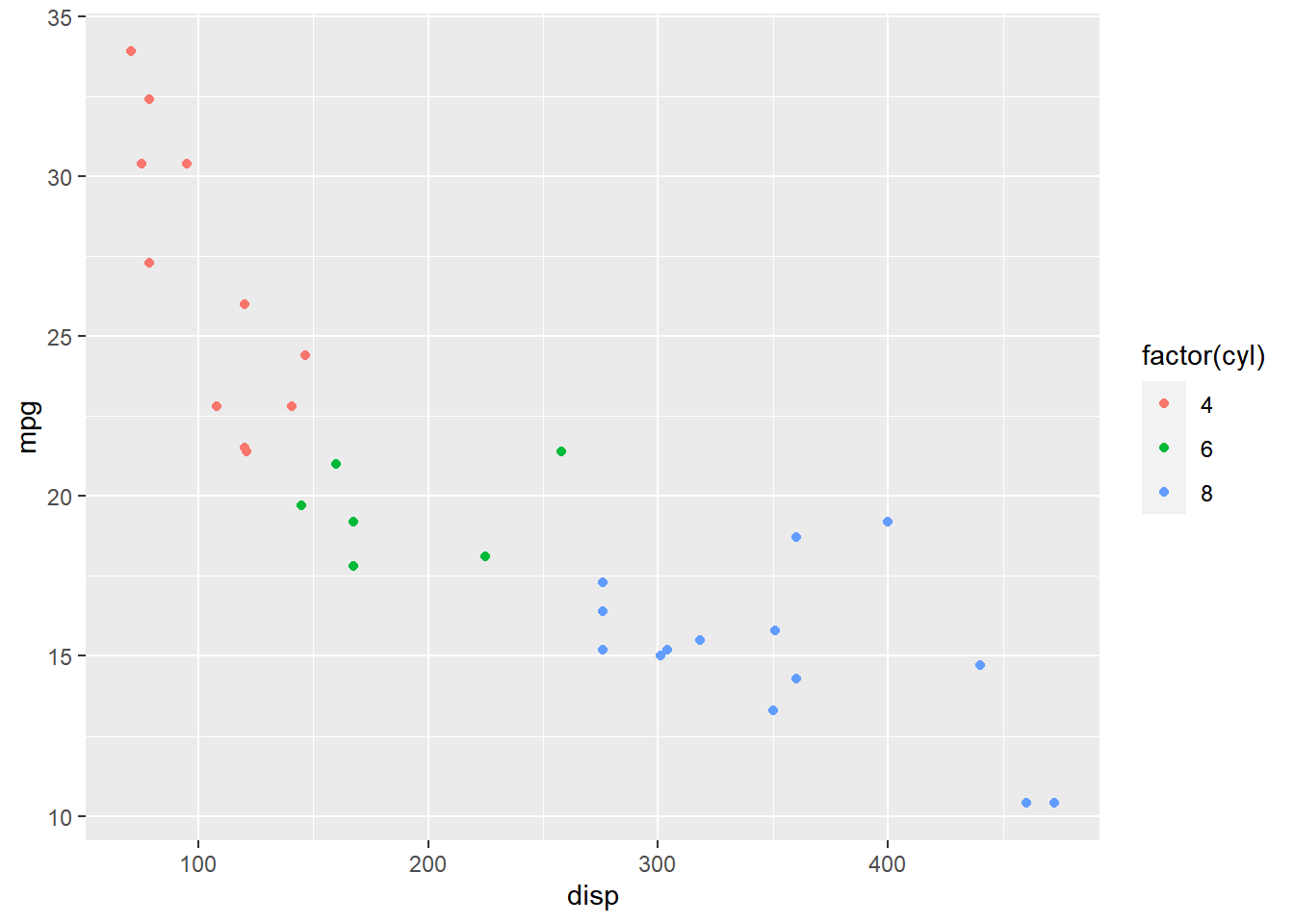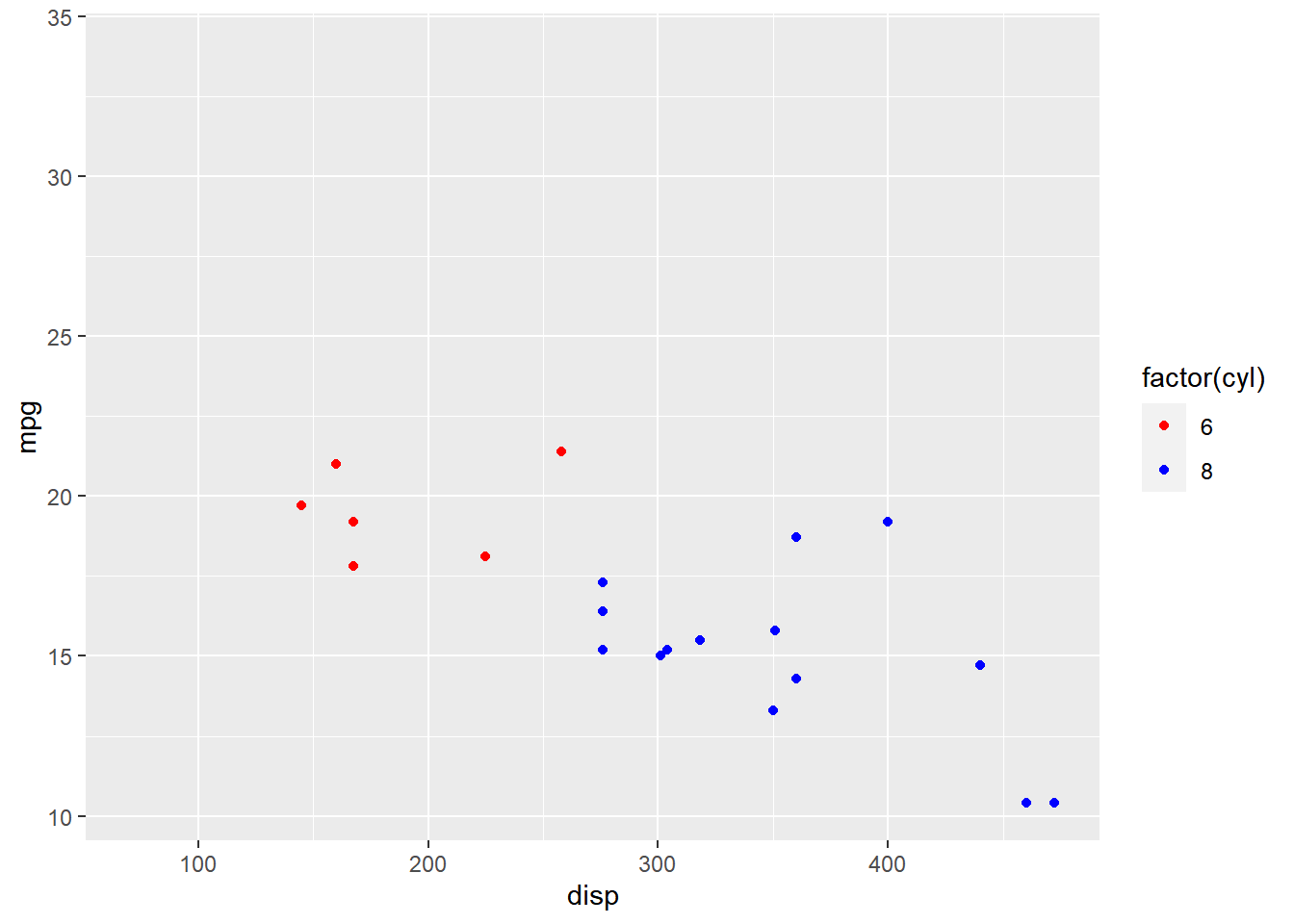Want to share your content on R-bloggers? click here if you have a blog, or here if you don't.

### Introduction

This is the 13th post in the series Elegant Data Visualization with ggplot2. In the previos post, we learnt how to modify the axis of plots. In this post, we will focus on modifying the appearance of legend of plots when the aesthetics are mapped to variables. Specifically, we will learn to modify the following when `color` is mapped to categorical variables:

• title
• breaks
• limits
• labels
• values

### Libraries, Code & Data

We will use the following libraries in this post:

All the data sets used in this post can be found here and code can be downloaded from here.

### Basic Plot

Let us start with a scatter plot examining the relationship between displacement and miles per gallon from the mtcars data set. We will map the color of the points to the `cyl` variable.

```ggplot(mtcars) +
geom_point(aes(disp, mpg, color = factor(cyl)))```As you can see, the legend acts as a guide for the `color` aesthetic. Now, let us learn to modify the different aspects of the legend.

### Values

To change the default colors in the legend, use the `values` argument and supply a character vector of color names. The number of colors specified must be equal to the number of levels in the categorical variable mapped. In the below example, `cyl` has 3 levels (4, 6, 8) and hence we have specified 3 colors.

```ggplot(mtcars) +
geom_point(aes(disp, mpg, color = factor(cyl))) +
scale_color_manual(values = c("red", "blue", "green"))```### Title

In the previous example, the title of the legend (`factor(cyl)`) is not very intuitive. If the user does not know the underlying data, they will not be able to make any sense out of it. Let us change it to `Cylinders` using the `name` argument.

```ggplot(mtcars) +
geom_point(aes(disp, mpg, color = factor(cyl))) +
scale_color_manual(name = "Cylinders",
values = c("red", "blue", "green"))```Now, the user will know that the different colors represent number of cylinders in the car.

### Limits

Let us assume that we want to modify the data to be displayed i.e. instead of examining the relationship between mileage and displacement for all cars, we desire to look at only cars with at least 6 cylinders. One way to approach this would be to filter the data using `filter` from dplyr and then visualize it. Instead, we will use the `limits` argument and filter the data for visualization.

```ggplot(mtcars) +
geom_point(aes(disp, mpg, color = factor(cyl))) +
scale_color_manual(values = c("red", "blue", "green"), limits = c(6, 8))
## Warning: Removed 11 rows containing missing values (geom_point).```As you can see above, `ggplot2` returns a warning message indicating data related to 4 cylinders has been dropped. If you observe the legend, it now represents only 4 and 6 cylinders.

### Labels

The labels in the legend can be modified using the `labels` argument. Let us change the labels to `Four`, `Six` and `Eight` in the next example. Ensure that the labels are intuitive and easy to interpret for the end user of the plot.

```ggplot(mtcars) +
geom_point(aes(disp, mpg, color = factor(cyl))) +
scale_color_manual(values = c("red", "blue", "green"),
labels = c('Four', 'Six', 'Eight'))```### Breaks

When there are large number of levels in the mapped variable, you may not want the labels in the legend to represent all of them. In such cases, we can use the breaks argument and specify the labels to be used. In the below case, we use the `breaks` argument to ensure that the labels in legend represent two levels (4, 8) of the mapped variable.

```ggplot(mtcars) +
geom_point(aes(disp, mpg, color = factor(cyl))) +
scale_color_manual(values = c("red", "blue", "green"),
breaks = c(4, 8))```### Putting it all together…

```ggplot(mtcars) +
geom_point(aes(disp, mpg, color = factor(cyl))) +
scale_color_manual(name = "Cylinders", values = c("red", "blue", "green"),
labels = c('Four', 'Six', 'Eight'), limits = c(4, 6, 8), breaks = c(4, 6, 8))```### Summary

In this post, we learnt to modify the following aspects of legends:

• title
• breaks
• limits
• labels
• values

### Up Next..

In the next post, we will learn how to modify legend when `fill` is mapped to variables.